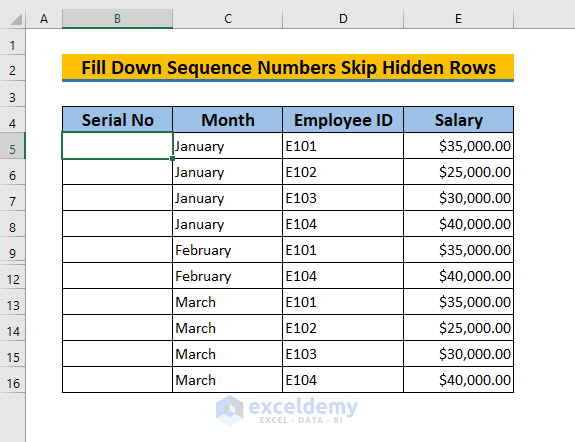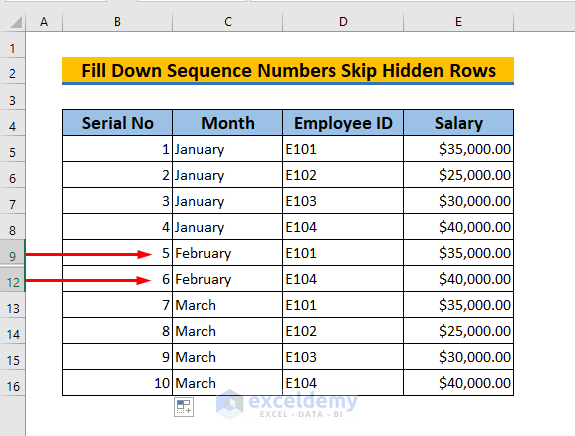# Excel Formulas to Fill Down Sequence Numbers Skip Hidden Rows

Get FREE Advanced Excel Exercises with Solutions!

Sometimes we need to hide some unnecessary rows in Excel to present the information. There we frequently face a problem filling down the sequence numbers skipping hidden rows. That’s why today we will going to discuss some methods on how to fill down sequence numbers skip hidden rows in Excel.

Here we took an Excel file having 4 columns: Serial No, Month, Employee ID, and Salary wherein rows 10, 11 and 12 are missing. We have to fill down the sequence numbers in column B skipping hidden rows.## 3 Ways to Fill Down Sequence Numbers in Excel Skip Hidden Rows

In this article, we will be going to discuss the top 3 methods on how to fill down sequence numbers skip hidden rows in Excel.

### 1. Using AGGREGATE Function to Fill Down Sequence Numbers Skip Hidden Rows in Excel

This one is the most convenient method to perform the fill down the sequence number skipping the hidden rows in Excel. To perform it using the AGGREGATE function, we need to follow the following steps:

👇 STEPS:

• You have to select cell B5

###• Then you have to apply the following formula:
`=(AGGREGATE(2,7,\$B\$4:B4))+1`
Formula Explanation

Here, 2 and 7 in (AGGREGATE(2,7,..) represent the nested SUBTOTAL and AGGREGATE function while ignoring the hidden rows and error values respectively in the range \$B\$4:B4 under which we want to start the sequence numbers and that’s why we need to add 1 with it.• After that, click ENTER and you will get 1 in cell B5.• Next, use Fill Handle to AutoFill the formula for the rest of the cells to perform the same operation for other cells in Excel.• Eventually, we will be able to fill down sequence numbers skip hidden rows as shown in the picture below.

###Read More: How to Use Autofill Formula in Excel

### 2. Fill Down Sequence Numbers Skip Hidden Rows in Excel Using SUBTOTAL Function

We can easily perform it by using the SUBTOTAL function too. To do so, we need to perform the following steps:

👇 STEPS:

• Select cell B5• Then apply the following formula:
`=SUBTOTAL(103,\$C\$5:C5)`
Formula Explanation

Here SUBTOTAL(103,\$C\$5:C5) represents the total numeric value in the range \$C\$5:C5 ,and using the first argument 103 refers to the COUNTA function while it counts numbers ignoring the hidden rows and error values.• After that, click ENTER and you will get 1 in cell B5.• Next, use Fill Handle to AutoFill the formula for the rest of the cells to fill down the sequence numbers skip hidden rows for other cells in Excel.• Eventually, we will be able to fill down sequence numbers skip hidden rows as shown in the picture below.

### 3. Using VBA Code to Fill Down Sequence Numbers Skip Hidden Rows

We can perform this fill down the sequence number skipping the hidden rows in Excel by using Visual Basic for Application (VBA) too. To do so, we have to follow the following methods:

👇 STEPS:

• First, select all cells in range B5:B16.• Go to Developer Tab >> Select Visual BasicThen there appears a new window. Next,

• Select Sheet4 (VBA) >> Go to Insert >> Select ModuleAfter that, there appears a Module Box there to write down your VBA codes. Then apply the following codes there:

``````Sub FillDownSequenceNumbers()
Dim Xrng As Range
Dim Ycell As Range
Dim ExcelTxt As String
Dim Xvl As Long
Dim I As Long
On Error Resume Next
Set Xrng = Application.InputBox("Select Range", "Fill Down Sequence Numbers", ExcelTxt, , , , , 8)
Set Xrng = Xrng.SpecialCells(xlVisible)
If Xrng Is Nothing Then Exit Sub
For Each Ycell In Xrng
Xvl = Xvl + 1
Ycell = Xvl
Next
End Sub``````• By saving it, press F5 to run this code. Next, there appears an InputBox to select range and you just need to click OK• Eventually, go to your Excel sheet and find the desired sequence numbers skip hidden rows as shown in Excel.

##Read more: How to Autofill Dates in Excel

## Practice Yourself

I’ve given a practice sheet in the workbook to practice these explained ways to fill down the sequence number skipping the hidden rows in Excel. You can download it from the link given above.## Conclusion

In this article, I have tried to explain 3 different ways to fill down the sequence number skipping the hidden rows in Excel. Last but not least, I will be extremely grateful if you comment down below any of your suggestions, ideas, or feedback.

## Related ArticlesFoyez Alam

Hello everyone, This is Foyez Alam. I have recently completed my MBA from Institute of Business Administration (IBA), University of Dhaka. Earlier I have completed my B.Sc in Leather Engineering from University of Dhaka. Now I am working as an Excel and VBA Content Developer in SOFTEKO. Through patience and hard work, I have a passion for learning new things and applying my knowledge to new situations. That's why I am here. Besides this, I love to interact with different people and I love to spend my spare time reading, watching movies, dancing, etc.

1. ReplyWhen I enter the aggregate the fill handle doesn’t work!

• ReplyHello WILL,
Thanks for your feedback. There are some reasons that are why you may have faced the problem. You can solve it by following the steps:

1. Maybe your Fill Handle tool is deactivated. To activate it, Click File > Options > Advanced > Enable fill handle and cell drag and drop.

2. The AGGREGATE function can work only for vertical ranges, not for horizontal ranges. So always apply it for vertical ranges and then the Fill Handle should work.

3. The AGGREGATE function is available since 2010, so if you are using an older version of Excel then it won’t work.

If the above solutions fail to rescue you then your issue is quite particular and that is difficult to find out without the file. So if you share your file with us then we hope, we could provide you with the exact solution.